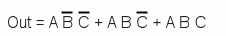# Complex gate creation

You cannot always find a standard gate that fit your needs. In these case, you have to do it yourself.

You must start with the truth table of the gate you need.

For example, let say a 3 inputs / 1 output system.

A Out 00001011

To find the equivalent logic circuit, you sum (OR) the input conditions of each output set to 1:

A B Out 00001011 A B C A B CA B C

Hence the gate formula that leads directly to the first solution (see the LogiFlash simulation beneath).This circuit can be simplified by using the formulas found in the previous pages:Hence the final solution.

You can see here a comparison of the two circuits, before and after the optimization. Hopefully, they work the same and give the same results.